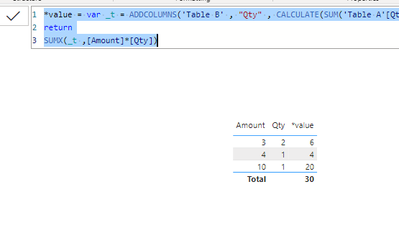cancel
Showing results for
Did you mean:Frequent Visitor

## Need Formula of DAX Calculation for Quantity by Amount

Hello,

I have the below data:

 Qty Amount Total 2 10 20 2 3 6 1 4 4

Total                                30 - Result Expecting in a new measure

I have Qty column in TableA and Amount column in TableB , I was creating a DAX calculation (A New Measure) in TableA where in i should be able to multiply TableA[Qty] * TableB[Amount]

I am trying to create a DAX caluclation for this where in i will get the total of that calculation as "30" shown in the table and display the total '30' into a CARD visual in Power BI.

Solution i tried:

TableANewMeasure = Sum('TableA'[Qty]) * Sum('TableB'[Amount])

I am a newbie so was trying to solve this simple issue. Thanks in Advance for your help.

Thank You,

TSN

1 ACCEPTED SOLUTIONCommunity Support

Hi , @TSN

According to your description, you want to calculate the sum of the [Quantity] * [Amount]. Right?

Here are the steps you can refer to :

(1)This is my test data:(2)We can click "New measure" and enter this:

``````*value = var _t = ADDCOLUMNS('Table B' , "Qty" , CALCULATE(SUM('Table A'[Qty])))
return
SUMX(_t ,[Amount]*[Qty])``````

(3)Then we can put this on the visual and we can meet your need:Thank you for your time and sharing, and thank you for your support and understanding of PowerBI!

Best Regards,

Aniya Zhang

If this post helps, then please consider Accept it as the solution to help the other members find it more quickly

10 REPLIES 10Community Support

Hi , @TSN

According to your description, you want to calculate the sum of the [Quantity] * [Amount]. Right?

Here are the steps you can refer to :

(1)This is my test data:(2)We can click "New measure" and enter this:

``````*value = var _t = ADDCOLUMNS('Table B' , "Qty" , CALCULATE(SUM('Table A'[Qty])))
return
SUMX(_t ,[Amount]*[Qty])``````

(3)Then we can put this on the visual and we can meet your need:Thank you for your time and sharing, and thank you for your support and understanding of PowerBI!

Best Regards,

Aniya Zhang

If this post helps, then please consider Accept it as the solution to help the other members find it more quicklyFrequent Visitor

@v-yueyunzh-msft  Thank You, This works! , Marking this as solution to this.

Thank You,Super User

Hi @TSN

NewMeasure =
SUMX (
SUMMARIZE (
TableA,
TableB[MatNbr],
TableB[Amount],
"@Qty", SUM ( TableA[Qty] )
),
[Amount] * [@Qty]
)Frequent Visitor

@tamerj1  This is not working. The Calculation Qty * Amount is coming as incorrect.Super User

Hi @TSN

What is the relationship between the two tables?Frequent Visitor

TableB::    1->*      ::TableA

[One - Many Relationship]

Thanks TSN,Super User

Via which column?Frequent Visitor

@tamerj1 : Via MatNbr column

Thank You,

TSNSuper User

@TSN First, please vote for this idea: https://ideas.powerbi.com/ideas/idea/?ideaid=082203f1-594f-4ba7-ac87-bb91096c742e

This looks like a measure totals problem. Very common. See my post about it here: https://community.powerbi.com/t5/DAX-Commands-and-Tips/Dealing-with-Measure-Totals/td-p/63376

Also, this Quick Measure, Measure Totals, The Final Word should get you what you need:
https://community.powerbi.com/t5/Quick-Measures-Gallery/Measure-Totals-The-Final-Word/m-p/547907

Also: https://youtu.be/uXRriTN0cfY
And: https://youtu.be/n4TYhF2ARe8

Become an expert!: Enterprise DNA
External Tools: MSHGQM
Latest book!:
Mastering Power BI 2nd EditionDAX is easy, CALCULATE makes DAX hard...Frequent Visitor

@Greg_Deckler , Thanks , Would you be more specific to this problem , As i am trying to calucate quantity * Amount = Total and the sum of that list of total. I saw the post as it is calculated based on the date.

As i am a newbie to this DAX , It would be much appreciated if you can provide a solution specific to my question -

Thanks,

TSN